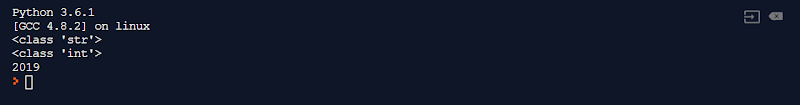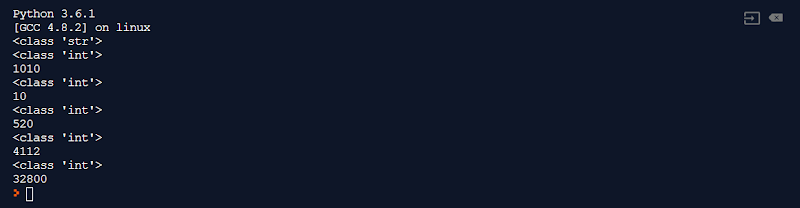In this tutorial we will see how to convert easily a Python Int to String and a Python String to int with straightforward examples using the Python built-in functions str() and int().Hi folks! Today, I will show you how to convert python Int to String and python String to Int so you can avoid "TypeErrors" problem when converting. Python language provides two built-in methods, the `str()` method and `int()` method. The `str()` function allows the conversion from Int type to a String type, the `int()` function takes a string object as an input and transforms it to an Integer object. Python has the type conversion functions that convert one data type to a different data type which is practical in today’s competitive programming. The `int()` is an out of the box function from Python that convert easily a string object into its integer value, it is invoked by passing  a string argument representing a number, and it returns the result as an integer value.

## Python Int to String Conversion

The type function is used to get the class type of any variable passed as an argument. Look at the following example.

``````# main.py

strNum = '123'
print(type(strNum))

pyc = 'Python type conversion'
print(type(pyc))

digit = 2019
print(type(digit))

numList = [1, 2, 4]
print(type(numList))

dictMovies = {1: 'John Wick', 2: 'Donnie Darko', 3: 'Zodiac'}
print(type(dictMovies))
``````

This code shows different class types and generates the following output on the Python console.To convert an integer value to a String, Python provides the `str()` function that you can use. Following are some examples that show a python conversion from Int to string.

This example shows an hexadecimal Int conversion into a string data type with python.

``````# main.py

intHex = 0x2a3
print(intHex)
print(type(intHex))
intHex = str(intHex)
print(intHex)
print(type(intHex))
``````

And this is the console output.Below you can see one more example that convert a base 10 int number to a String value with Python.

This code produces the following output.

``````# main.py
number = 2019
print(number)
print(type(number))
number = str(number)
print(number)
print(type(number))
``````## Python String to Int Conversion

To be able to convert a string representing a number value to an Int in Python, you will need to use the `int()` buil-in function that returns the constructed integer from a number or string passed as an argument. The example below demonstrates how to proceed.

``````# main.py

strNum = '2019'
print(type(strNum))

convertedInt = int(strNum)
print(type(convertedInt))
print(convertedInt)
``````

This code gives the following output.As you can see, we converted the Python String to int easily using the `int()` function.

## Convert String to Int from Different Base with Python

The `int()` function takes another argument named base, it is used only if the first argument representing the number is a String. The base argument specifies if the int belongs to different number base other than the default base 10. However, one more thing you need to consider is that the result returned by the Python `int()` function is always in base 10.

The base argument should be a number between 2 to 32. Have a look at the following example.

``````# main.py

strNum = '1010'
print(type(strNum))

convertedInt = int(strNum)
print(type(convertedInt))
print(convertedInt)

convertedInt2 = int(strNum, base=2)
print(type(convertedInt2))
print(convertedInt2)

convertedInt8 = int(strNum, base=8)
print(type(convertedInt8))
print(convertedInt8)

convertedInt16 = int(strNum, base=16)
print(type(convertedInt16))
print(convertedInt16)

convertedInt32 = int(strNum, base=32)
print(type(convertedInt32))
print(convertedInt32)
``````

This code generates the following output.## The ValueError Exception

The frequent ValueError Exception may be raised during the conversion process from String to Int, for the simple reason that the string value passed to the `int()` function as an input does not correspond to a correct number. For example, if you try to convert a string containing an hexadecimal value without specifying the base 16 argument in the `int()` function you will get the ValueError Exception.

Let’s see this in action in the following example.

``````# main.py

strHex = "0x11"
print(type(strHex))
convertedInt = int(strHex)
print(convertedInt)
``````

The above code will give the following result with a ValueError exception.## Conclusion

In this tutorial we have seen how to convert easily a Python Int to String and a String to int with straightforward examples using the Python built-in functions `str()` and `int()`. I hope you learned something today and stay tuned for more to come.

#python #pythonProgramming #pythonIntToString #pythonDataConversionName

Angular,7,Angular 8,1,Best Practices,1,Design,1,Firebase,1,Ionic,1,Java,5,Nodejs,3,Python,1,Restful API,1,Software Development,1,Spring,3,Spring Batch,1,Spring Boot 2,1,Typescript,1,Web Development,1,
ltr
item
Programming Tutorials, News and Reviews: Converting Python Int to String and Python String to Int
Converting Python Int to String and Python String to Int
In this tutorial we will see how to convert easily a Python Int to String and a Python String to int with straightforward examples using the Python built-in functions str() and int().
https://3.bp.blogspot.com/-rm2Di_p9-Jk/XQ40NlPgvzI/AAAAAAAAATI/6HT9c4GyQ38HWqb_NzNDvoid8MOQ68CuwCLcBGAs/s200/Converting%2BPython%2BInt%2Bto%2BString%2Band%2BPython%2BString%2Bto%2BInt.jpg
https://3.bp.blogspot.com/-rm2Di_p9-Jk/XQ40NlPgvzI/AAAAAAAAATI/6HT9c4GyQ38HWqb_NzNDvoid8MOQ68CuwCLcBGAs/s72-c/Converting%2BPython%2BInt%2Bto%2BString%2Band%2BPython%2BString%2Bto%2BInt.jpg
Programming Tutorials, News and Reviews